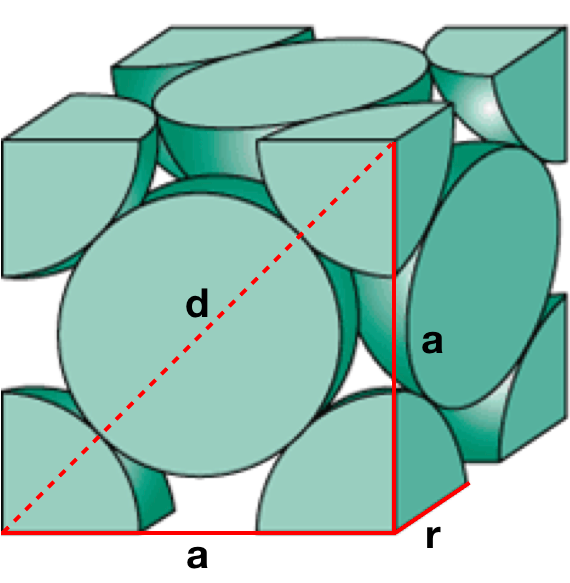# Problem: Pd crystallizes with a face-centered cubic unit cell. It has a density of 6.00 g/cm3 and a radius of 138 pm and a FW of 106.42 g/mol. Use these data to estimate Avagadro’s number.A. 6.0 x 1023B. 5.9 x 1023C. 3.1 x 1023D. 11.9 x 1023E. 6.0 x 1024

###### FREE Expert Solution

We’re being asked to calculate the Avogadro's number using the radius, density and formula weight of Pd metal

Recall that Avogadro's number is given by:

In this case, we have to find the number of atoms in an FCC crystal and the number of moles present using the provided data

face-centered cubic lattice contains an atom in each of the face of the cube and an atom in each of the corners. It looks like this:98% (254 ratings)###### Problem Details

Pd crystallizes with a face-centered cubic unit cell. It has a density of 6.00 g/cm3 and a radius of 138 pm and a FW of 106.42 g/mol. Use these data to estimate Avagadro’s number.

A. 6.0 x 1023

B. 5.9 x 1023

C. 3.1 x 1023

D. 11.9 x 1023

E. 6.0 x 1024